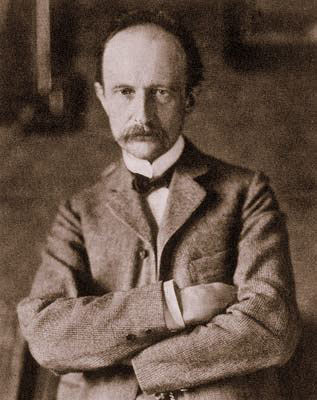The PLANCK RELATION andthe MASS OF THE PHOTON by Miles MathisThe Planck relation (or Planck-Einstein Relation) is just an equation relating the energy of a moving particle to its frequency, via the de Broglie wave. The particle does not have to be a photon; it can be any quantum, like an electron. E=hf Where h is Planck's constant. However, since I have shown that Planck's constant is hiding the mass of the photon, we may now unwind this equation, finding much more information buried beneath it. Using simple mechanical postulates, I have calculated that the mass of the infrared photon is h/2,400. To find this, I simply used G to scale down from the proton mass. You can also use the Dalton, 1821, to find this same mass. Simply cube the Dalton and invert it. The Dalton is an outcome of spin mechanics, and the photon is three levels below the proton and two levels below the electron. Using the Dalton, you will get 2,400, but using G will require you multiply by an additional 2.5. This is because G is a scale for size, not mass, and the density of the photon is not equal to the density of the proton. If we apply these new findings to the Planck relation, we see that the relation is between mass and energy, just like Einstein's equation E=mc2. And this means that the dimensions of h have been hiding something. Planck's constant is given complex dimensions to account for the transform to f, but if h contains a photon mass, then we are really transforming E into a mass, a frequency, and x: E = xmc f Let us apply the equation to the photon itself, to begin with. In that case x = cλE = mc cλfcλ = 2,400λ = 8 x 10-6mWhich is the infrared photon I chose to begin with.E = mc cλf = mc2Yes, Planck's relation is just a restatement of Einstein's equation, but the two together are used to hide the mass of the photon. The standard model forbids you from applying Einstein's equation to the photon, or from seeking this photon mass in the Planck relation. Why? Because that would mess up their gauge math.We can do the same thing for the electron: E = ½ me vλf = ½ mev2h = ½ me vλ The mass of the electron is 3.3 x 106 times greater than the mass of the infrared photon, so vλ must be much less than cλ. vλ = 2h/m = .00146 In the famous Davisson-Germer experiment of 1927, electrons were fired at a crystalline nickel target. We are told they were slow-moving, but are not given a velocity. We may now calculate it directly. The wavelength was measured from the experiment to be .165nm, which makes the velocity of the electrons 8.8 x 106m/s or .03c. Not so slow. I think you really have to ask why the standard model refuses to write the equations this way. Why do they refuse to show the simple mechanics and math I have shown here? The answer, again, is to save their gauge math, a math that already contains its own fields and symmetries. They have tried to match nature to the symmetries, but that has never worked for them. I would suggest they try to match their math to nature, rather than nature to math. They have already had to break their gauge symmetries to match experiments. If the gauge symmetries require breaking, then the matrices must have been wrong to start with. And if that is so, then the gauge math should be ditched as a whole. I cannot fathom why physicists are so in love with tools that do not work. It is as if they have a pathological obsession for the patches themselves. The modern physicist is like a carpenter in love with a golden hammer whose handle is cracked. The gold is too soft to work, and the head flies of with every swing; but the carpenter must use it and nothing else, since he has convinced himself that the shine of it is what generates his business. Addendum [February 2010]: we can use the above math to discover what quantum Planck's constant is really hiding. I have said that Planck's constant is hiding the mass of the photon, but since photons have different energies, we may ask which photon it is hiding. Well, we can see from above that h = mcλ. So we just make cλ = 1, and we will have h = m, you see. The photon that has a wavelength of 1/c is a photon with a wavelength of 3.3 x 10-9m, which is an X-ray. Since current physics is using h as the quantum of action, they must be using the X-ray as a quantum. This is not logical. It is much more logical to use the charge photon as a quantum. We should be using the infrared photon as the field quantum, not the X-ray. If this paper was useful to you in any way, please consider donating a dollar (or more) to the SAVE THE ARTISTS FOUNDATION. This will allow me to continue writing these "unpublishable" things. Don't be confused by paying Melisa Smith--that is just one of my many noms de plume. If you are a Paypal user, there is no fee; so it might be worth your while to become one. Otherwise they will rob us 33 cents for each transaction.